# R S Aggarwal Solutions for Class 10 Maths Chapter 6 Coordinate Geometry Exercise 6C

R S Aggarwal Solutions for Class 10 Maths Chapter 6 exercise 6c is available here. These R S Aggarwal solutions are prepared by subject experts to help students clear their doubts. The concepts are explained using step by step approach. This exercise is based on topic area of a triangle using vertex coordinates. Students can download Class 10 Maths Chapter 6 R S Aggarwal Solutions and start practicing offline.

## Download PDF of R S Aggarwal Solutions for Class 10 Maths Chapter 6 Coordinate Geometry Exercise 6C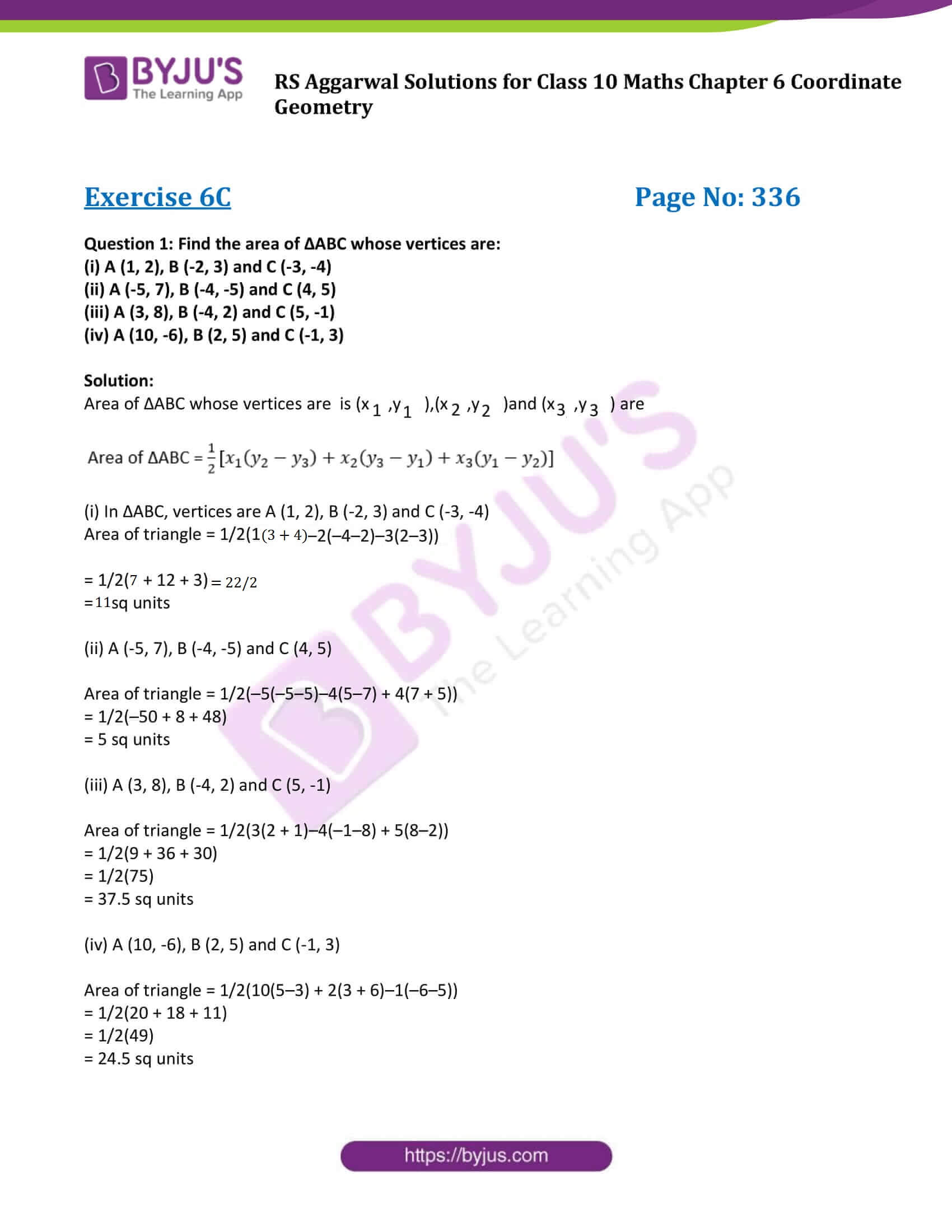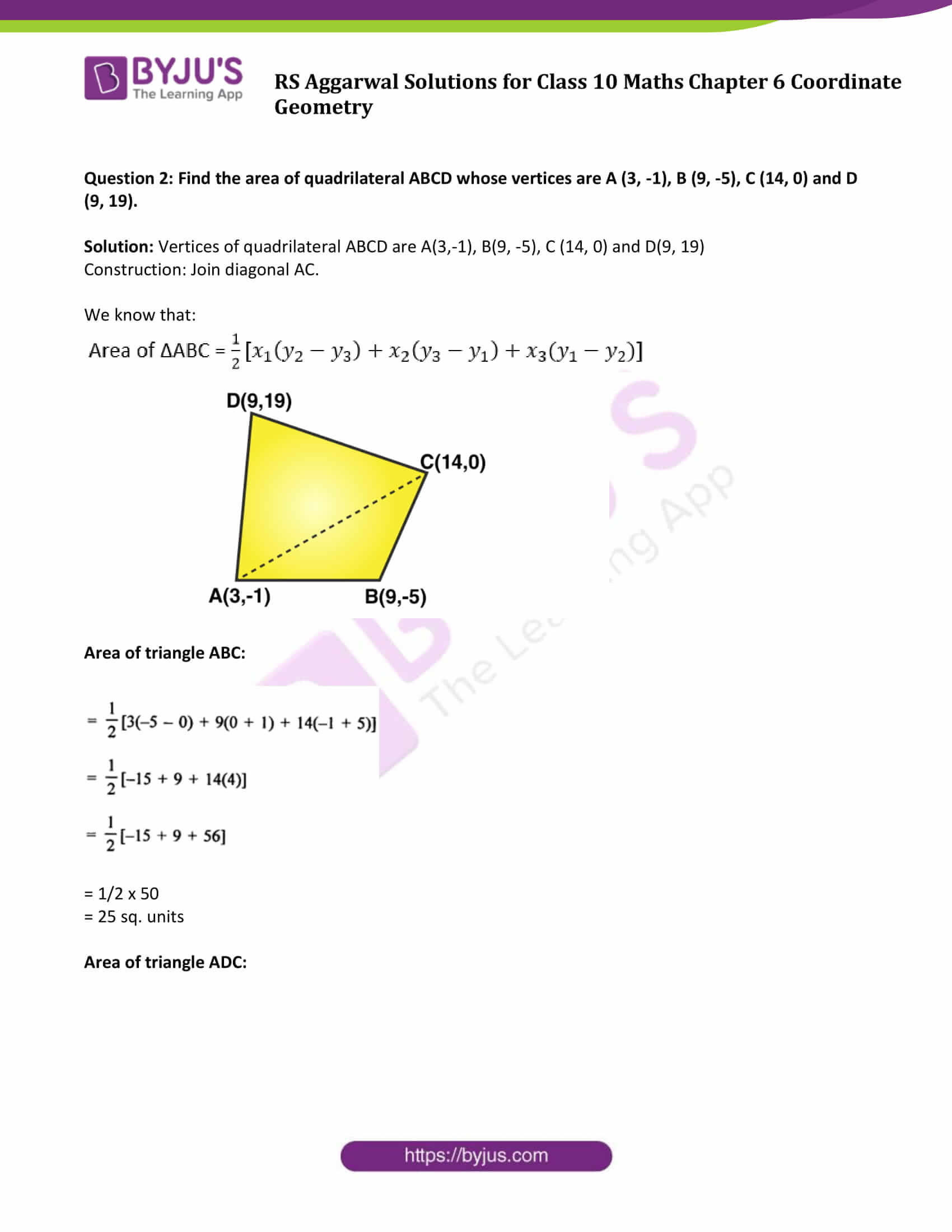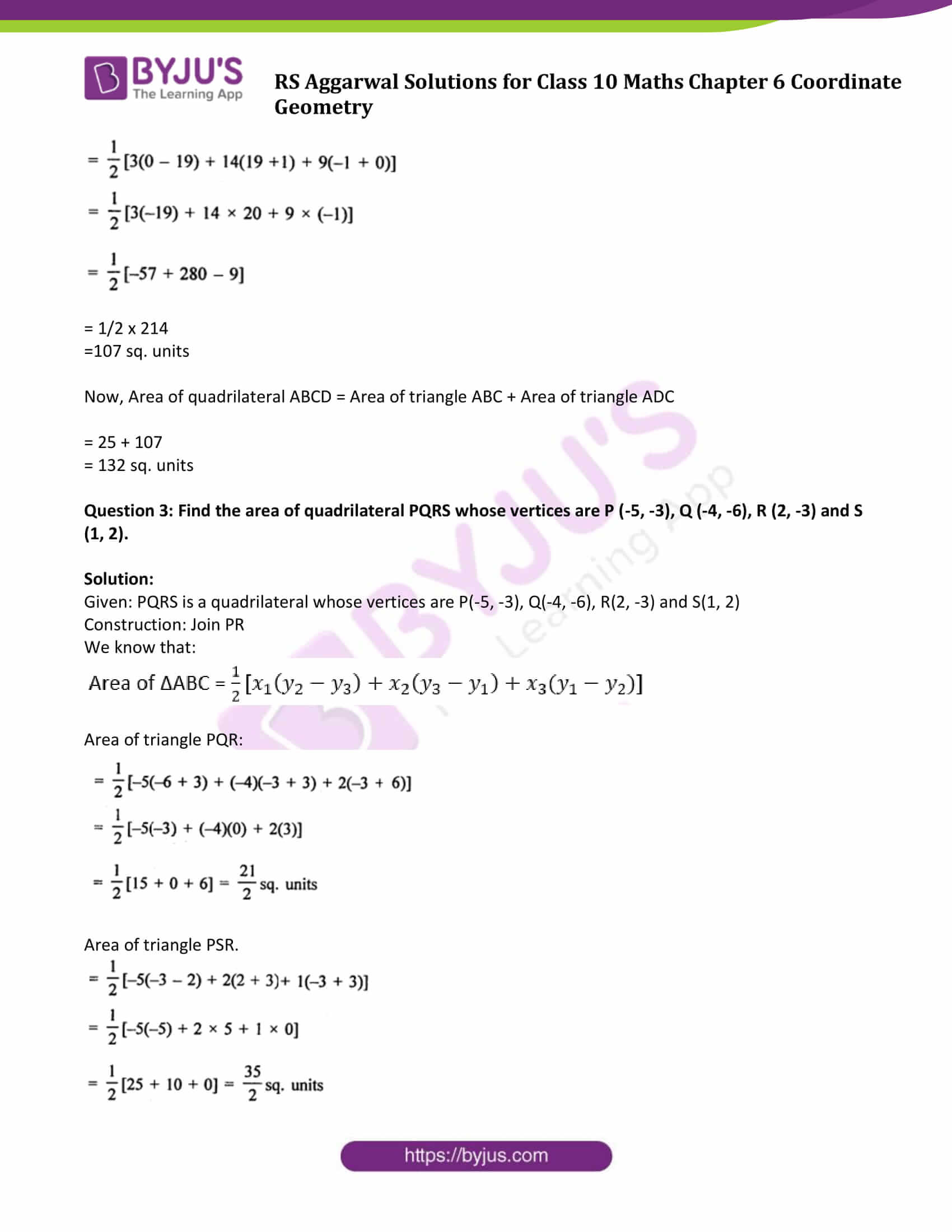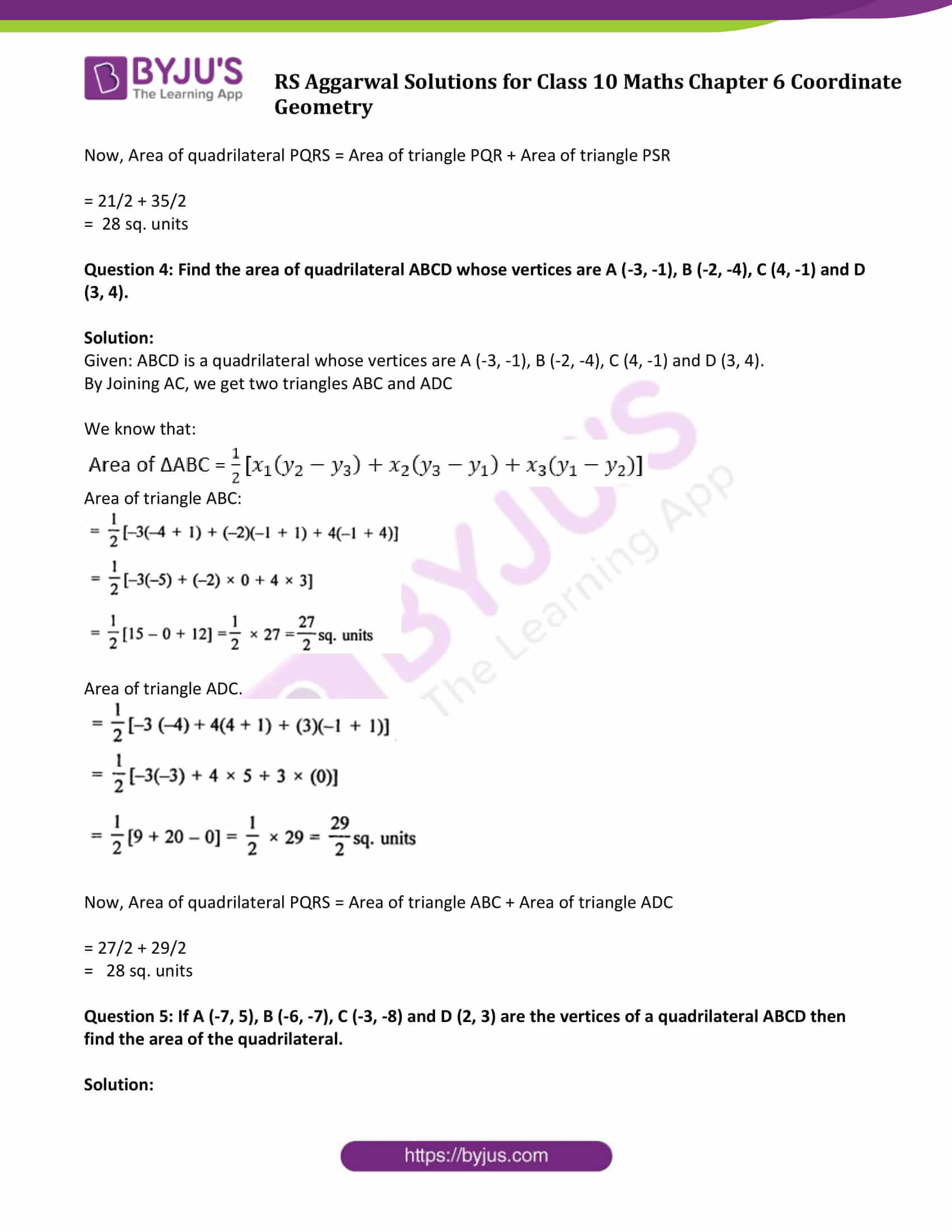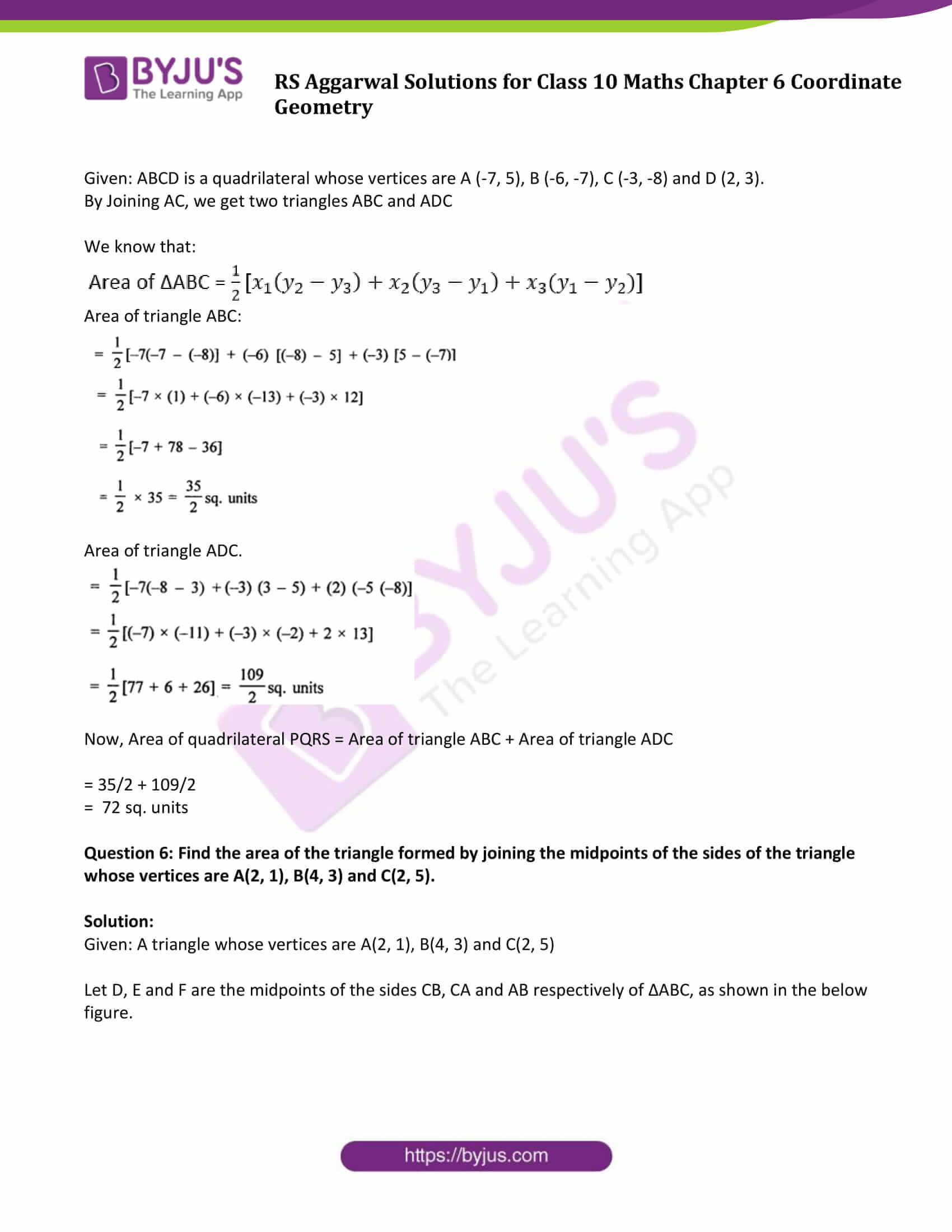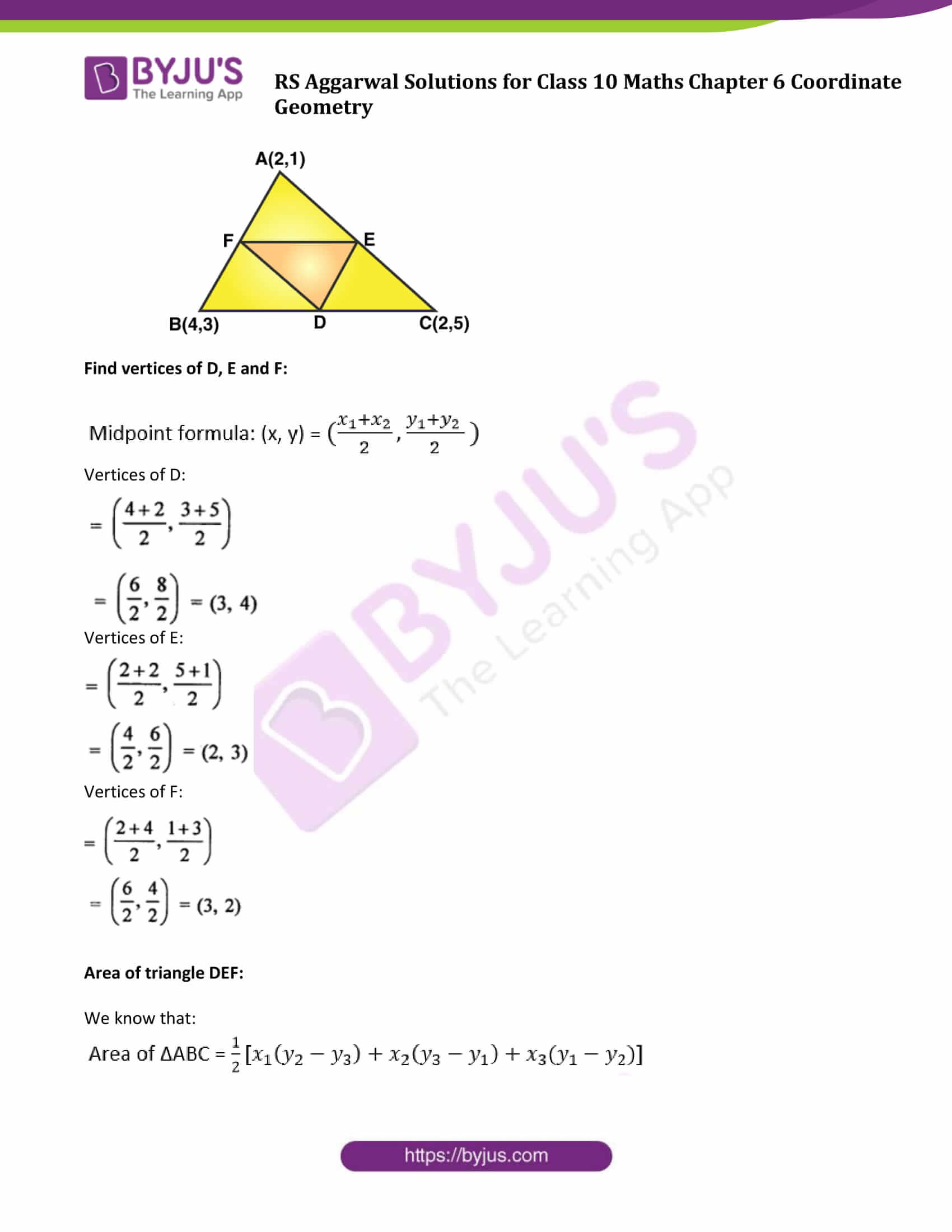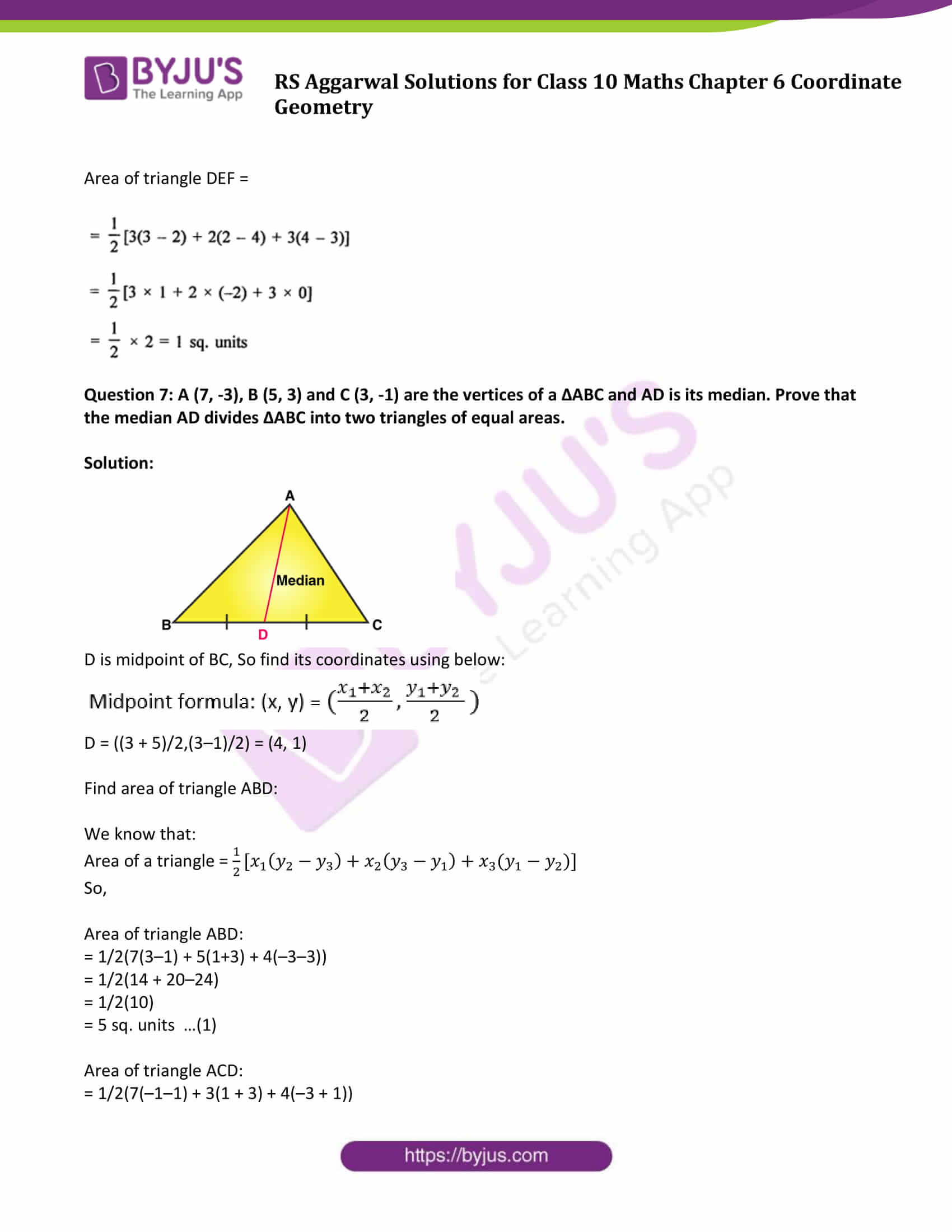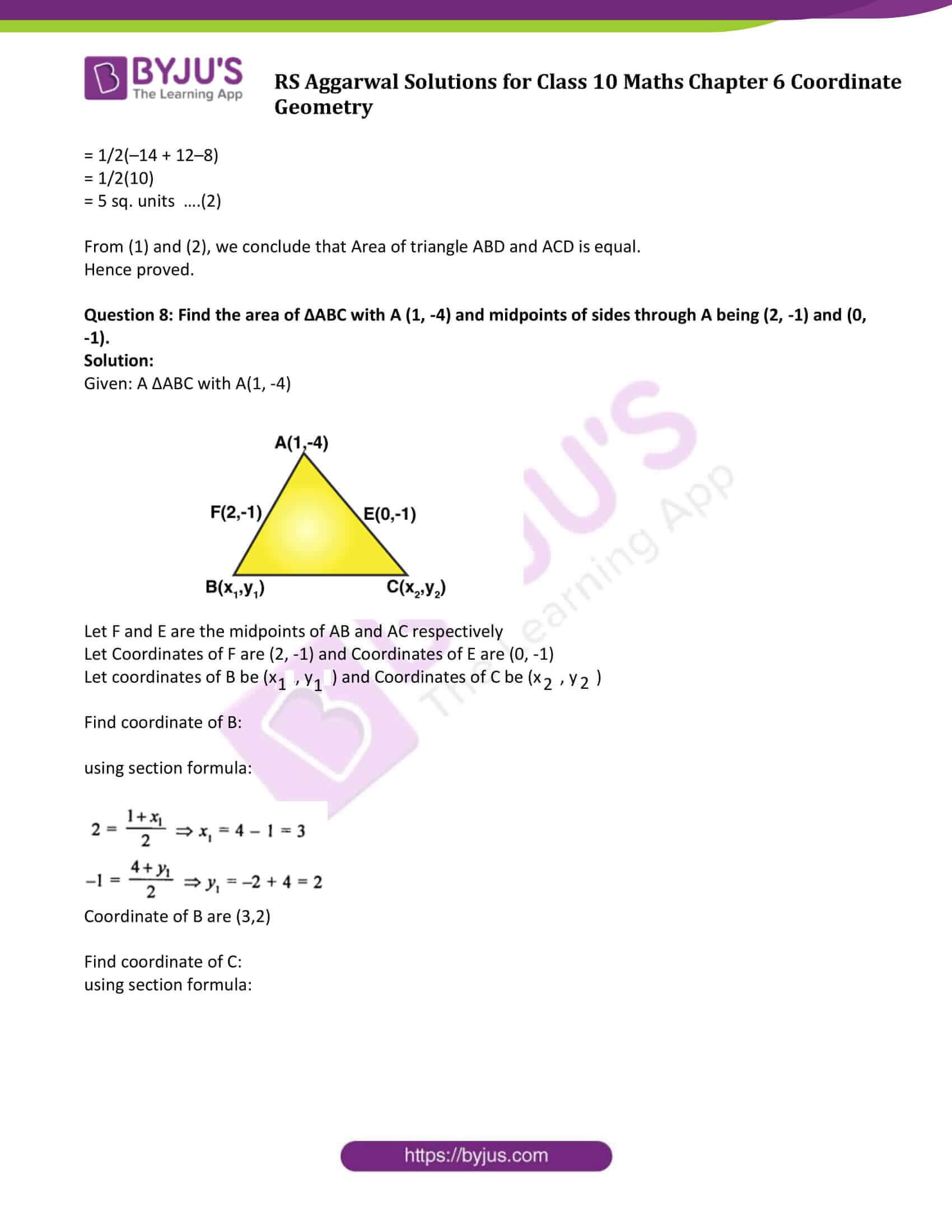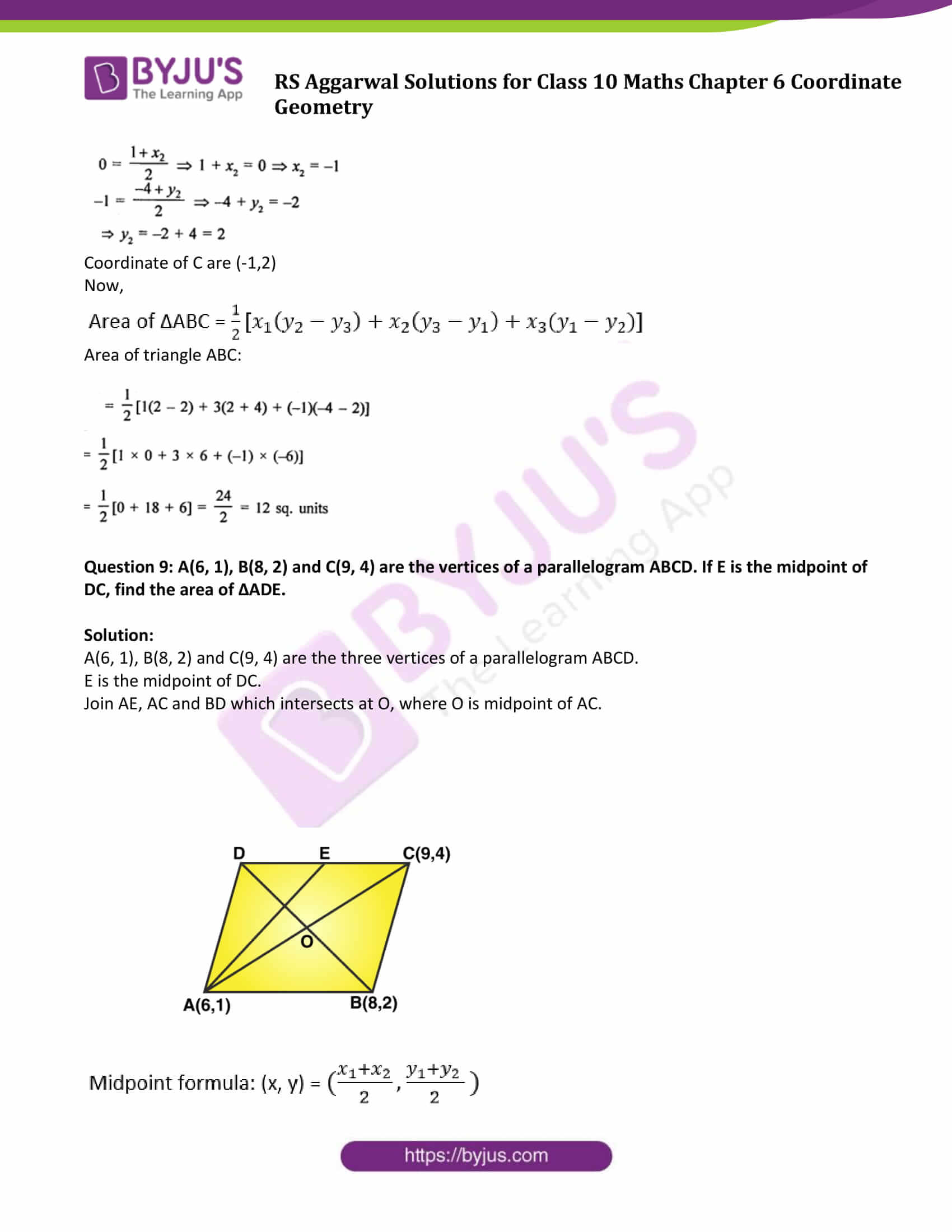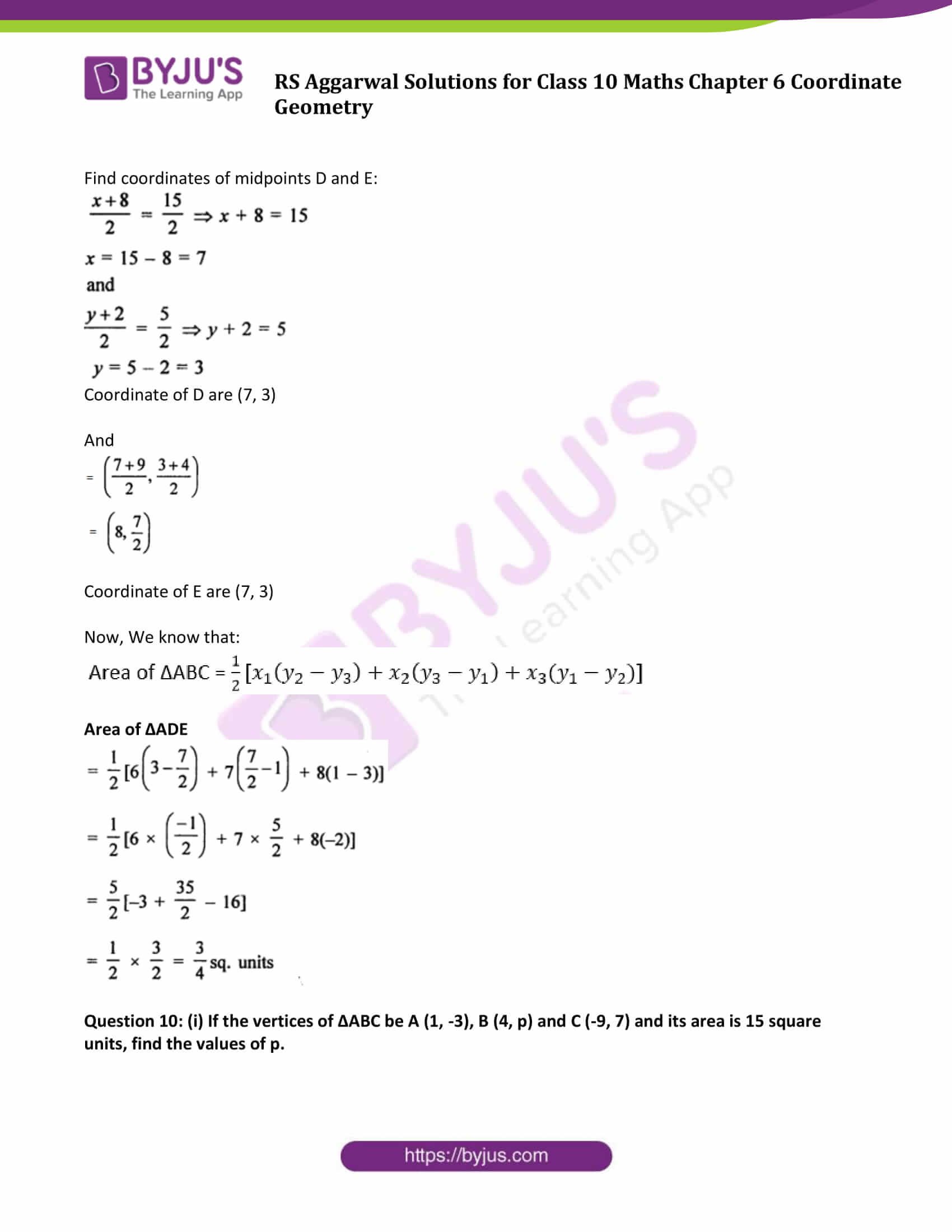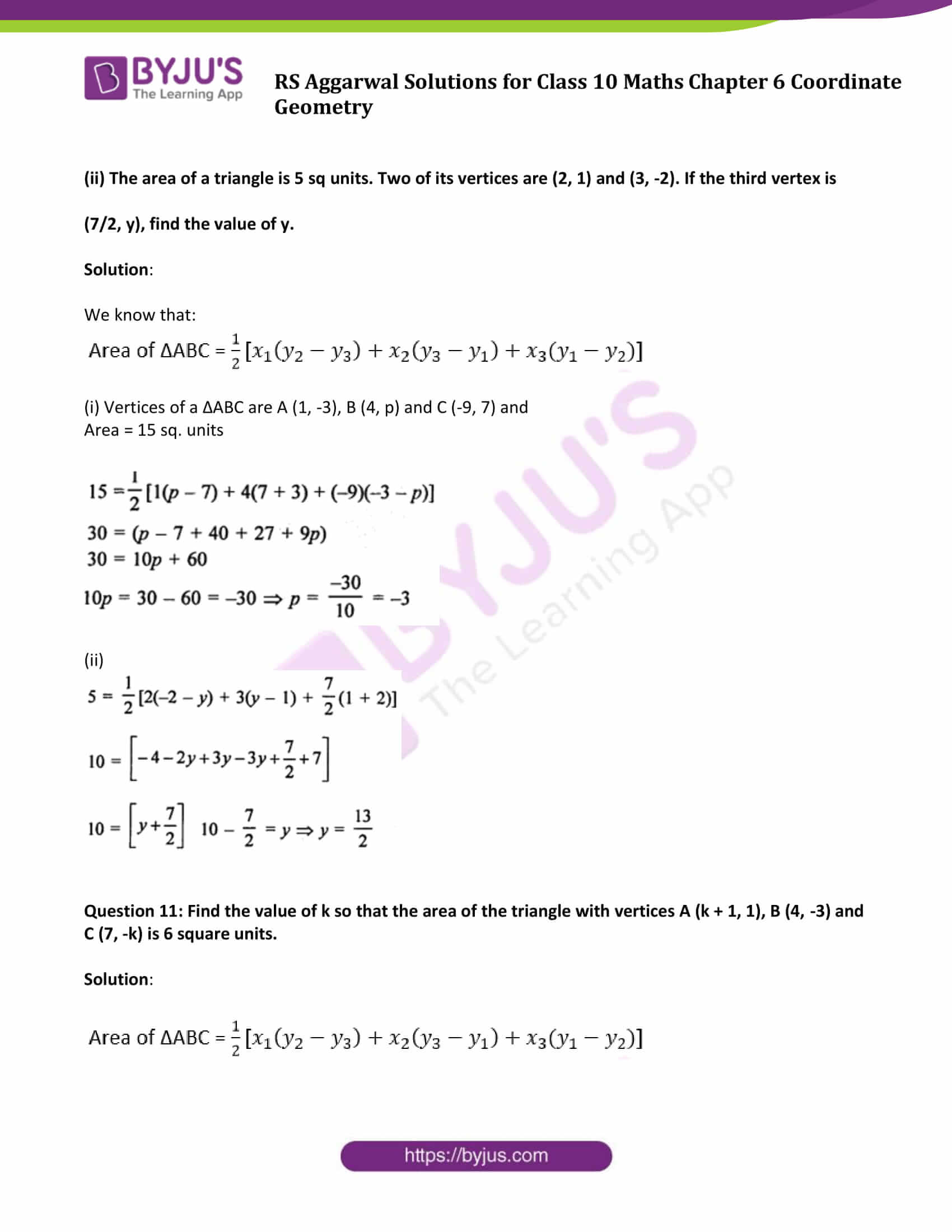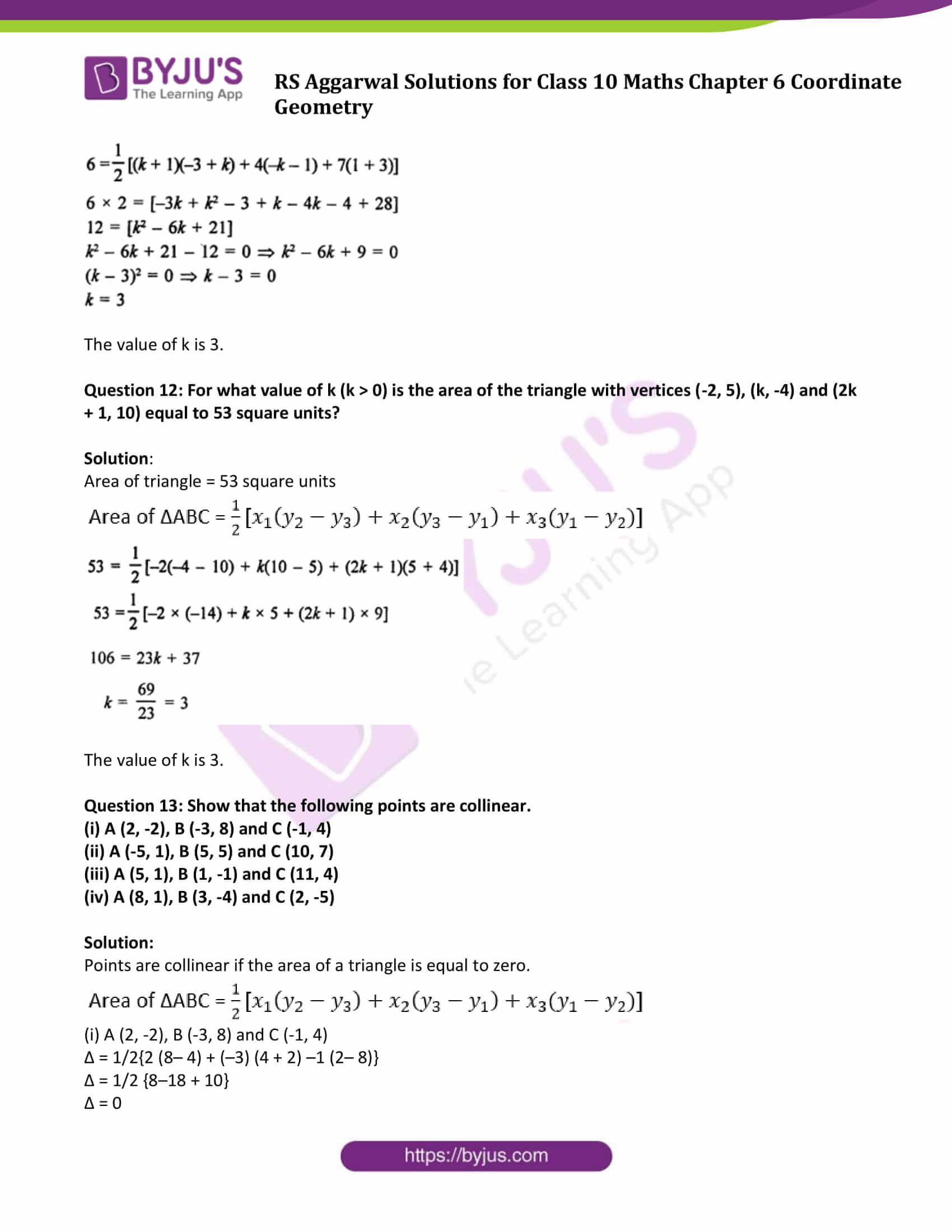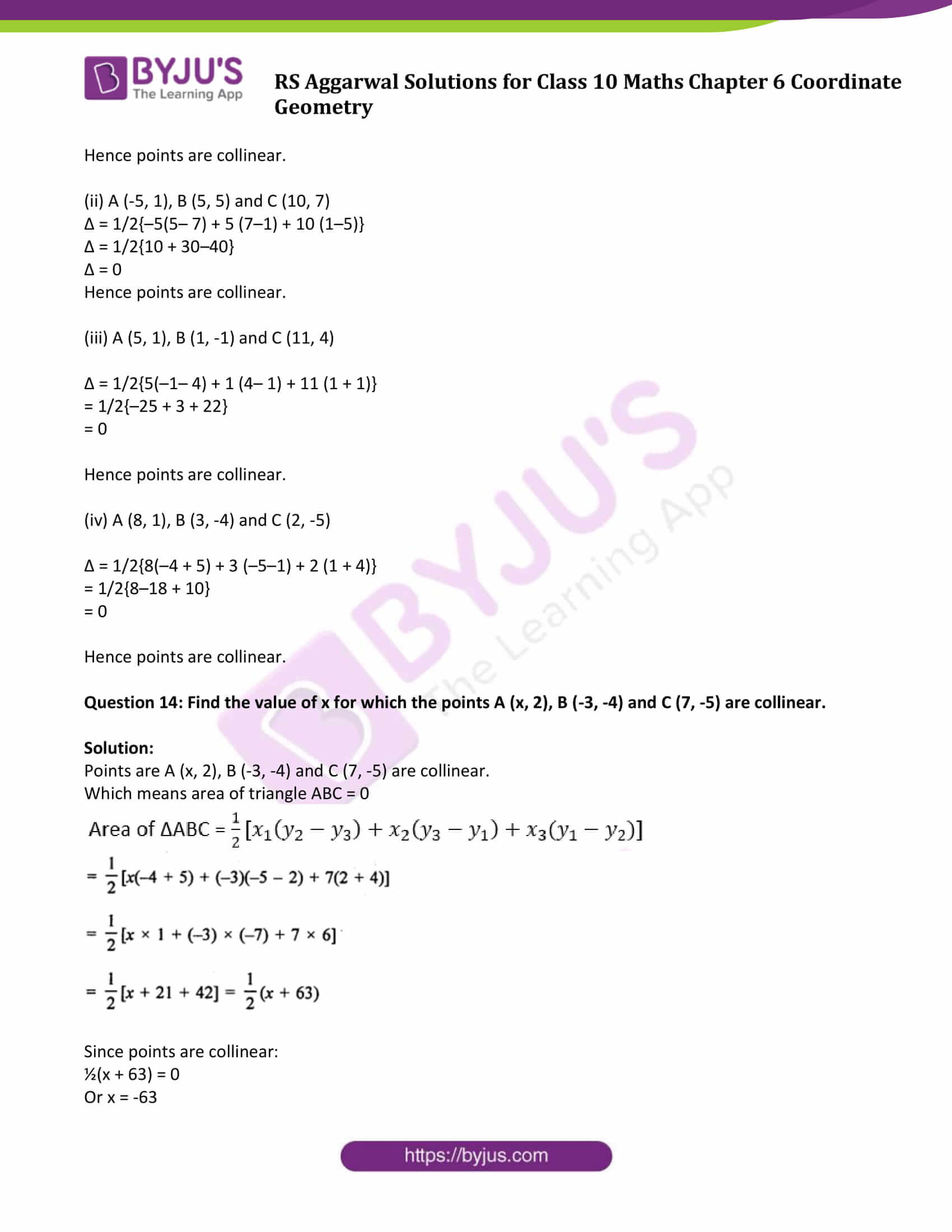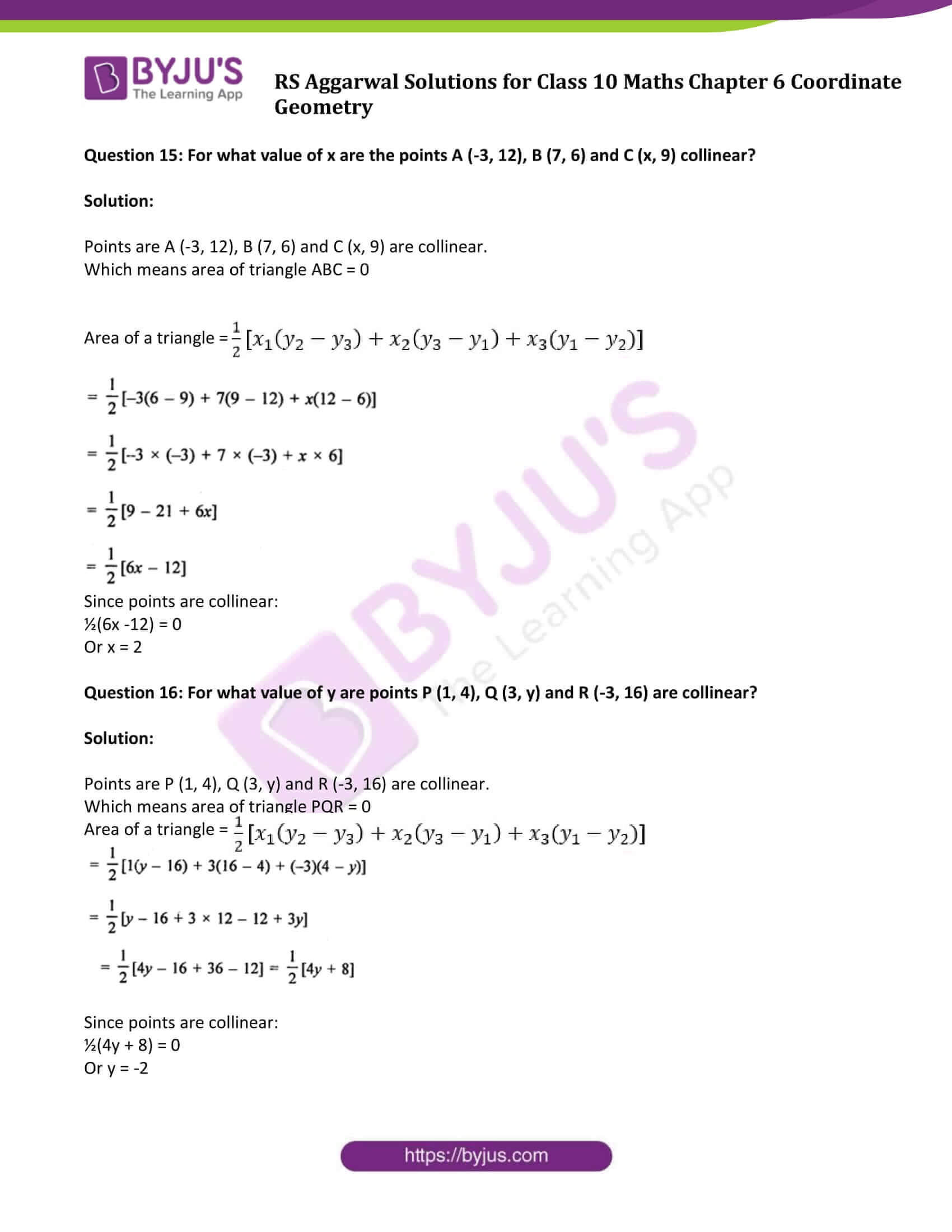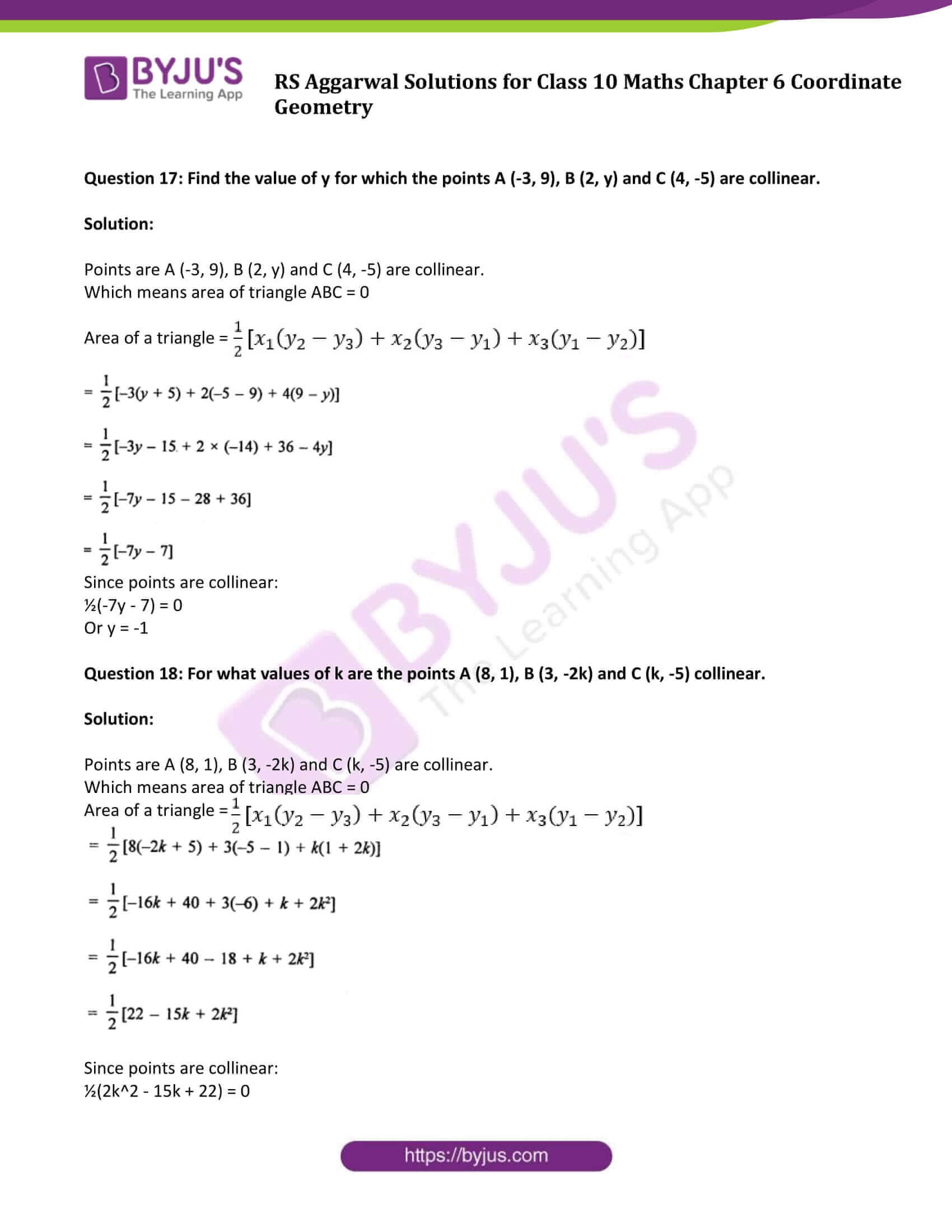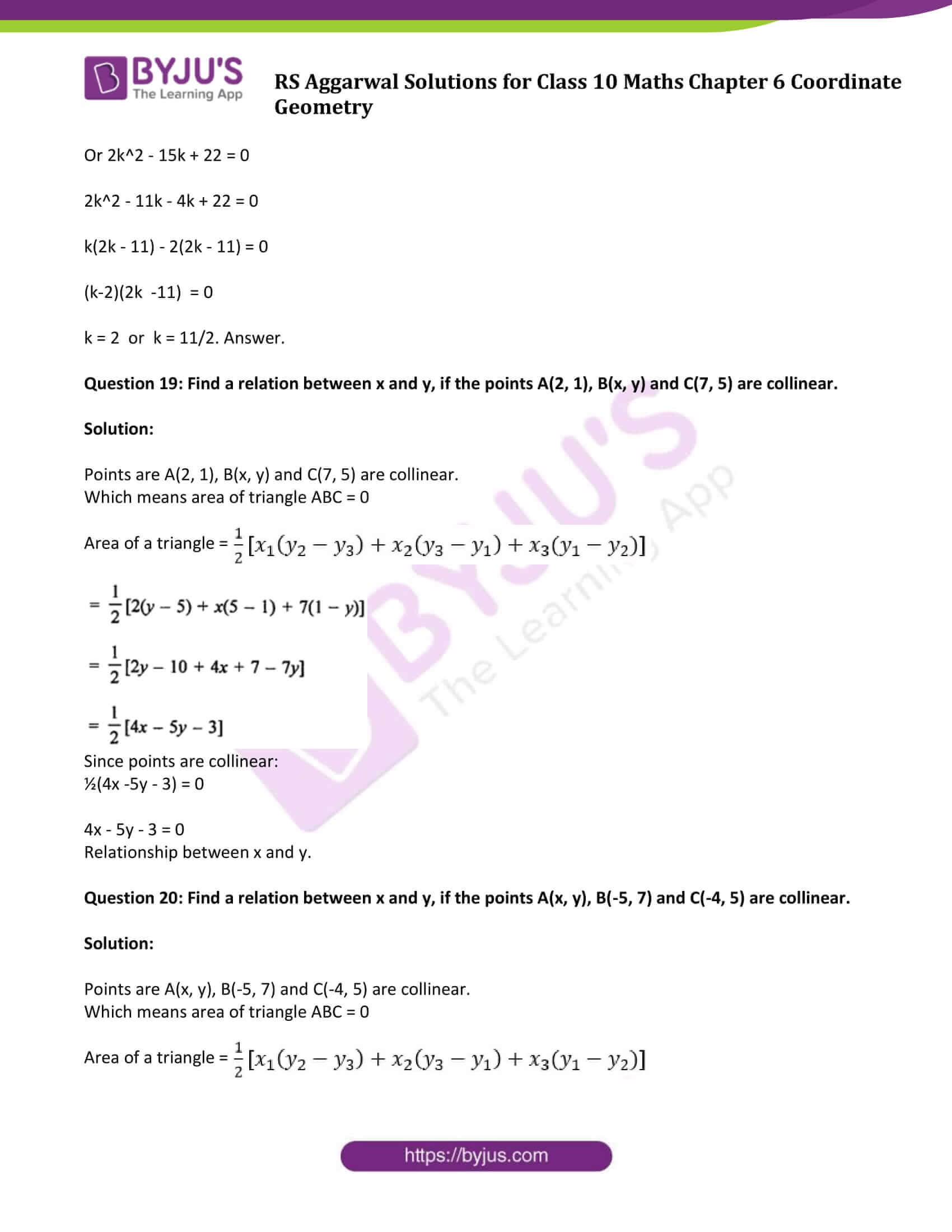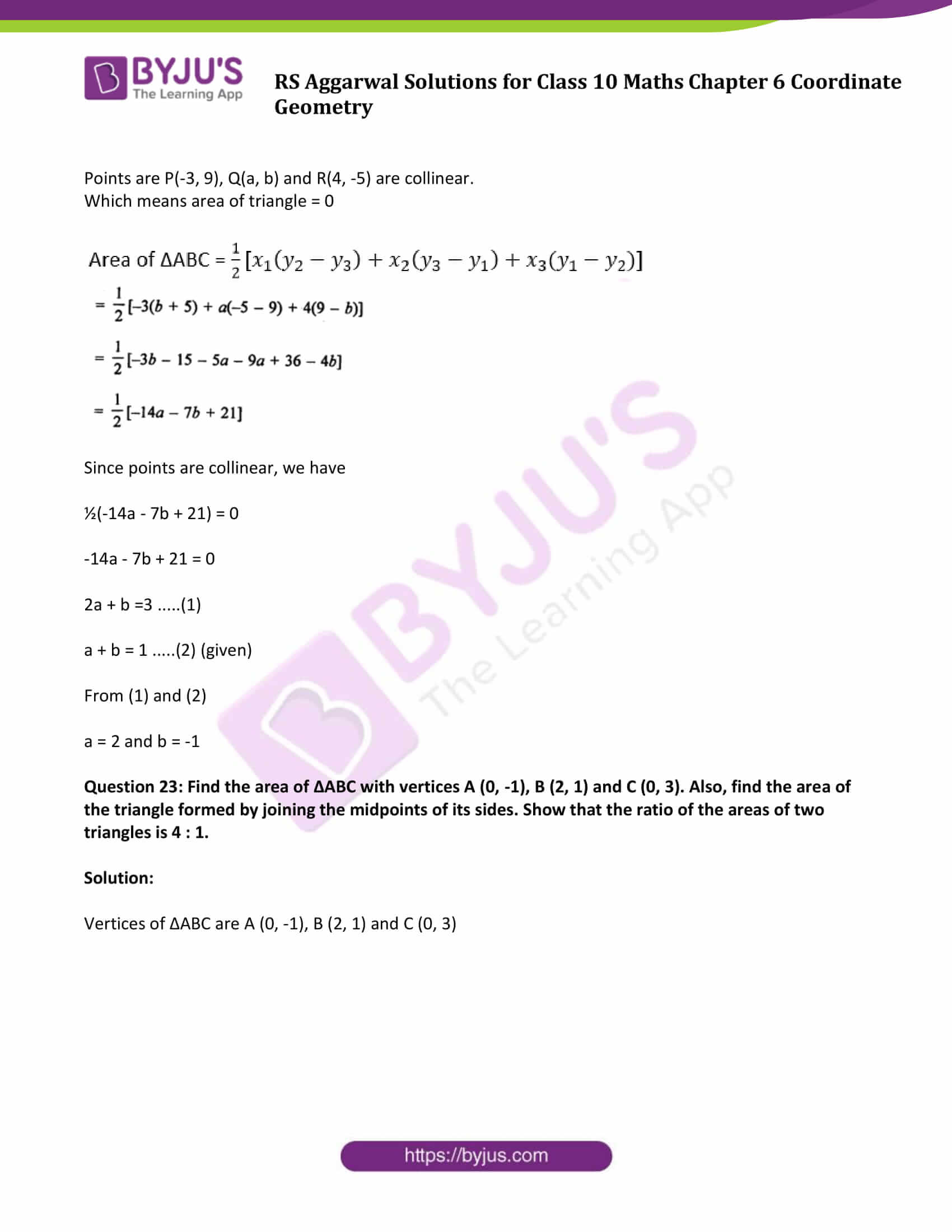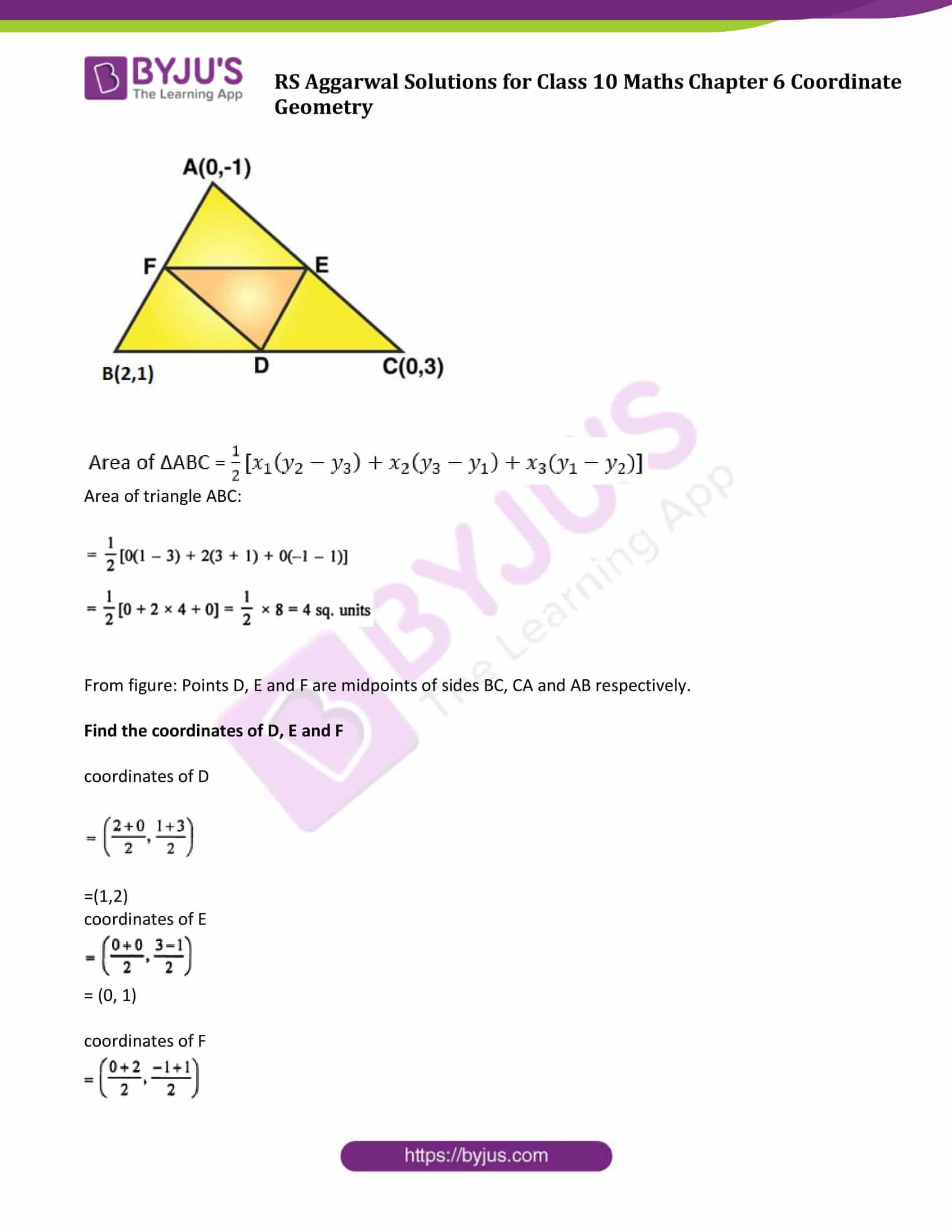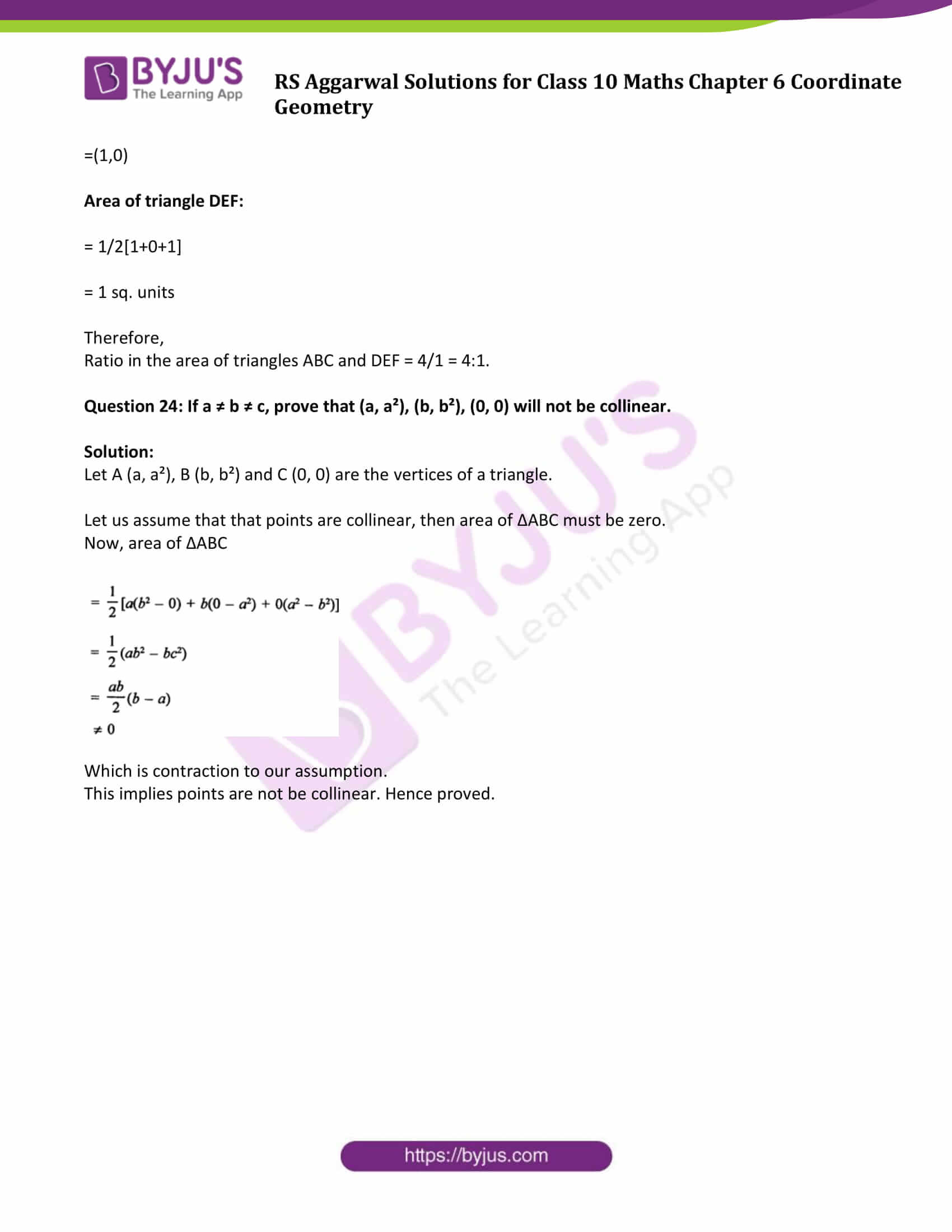### Access other exercise solutions of Class 10 Maths Chapter 6 Coordinate Geometry

Exercise 6A Solutions: 20 Questions (20 Short Answers)

Exercise 6B Solutions: 20 Questions (20 Short Answers)

Exercise 6D Solutions: 17 Questions (12 Short Answers & 5 Long Answers )

## Exercise 6C Page No: 336

Question 1: Find the area of ∆ABC whose vertices are:

(i) A (1, 2), B (-2, 3) and C (-3, -4)

(ii) A (-5, 7), B (-4, -5) and C (4, 5)

(iii) A (3, 8), B (-4, 2) and C (5, -1)

(iv) A (10, -6), B (2, 5) and C (-1, 3)

Solution:

Area of ∆ABC whose vertices are is (x1,y1 ),(x2,y2 )and (x3,y3 ) are

$Area of \bigtriangleup ABC=\frac{1}{2}\left [ x_{1}\left ( y_{2} – y_{3}\right ) + x_{2} \left( y_{3} – y_{1}\right )+ x_{3}\left( y_{1} – y_{2}\right )\right ]$

(i) In ∆ABC, vertices are A (1, 2), B (-2, 3) and C (-3, -4)

Area of triangle = 1/2(1(3 + 4)–2(–4–2)–3(2–3))

= 1/2(7 + 12 + 3) = 22/2

= 11 sq units

(ii) A (-5, 7), B (-4, -5) and C (4, 5)

Area of triangle = 1/2(–5(–5–5)–4(5–7) + 4(7 + 5))

= 1/2(–50 + 8 + 48)

= 5 sq units

(iii) A (3, 8), B (-4, 2) and C (5, -1)

Area of triangle = 1/2(3(2 + 1)–4(–1–8) + 5(8–2))

= 1/2(9 + 36 + 30)

= 1/2(75)

= 37.5 sq units

(iv) A (10, -6), B (2, 5) and C (-1, 3)

Area of triangle = 1/2(10(5–3) + 2(3 + 6)–1(–6–5))

= 1/2(20 + 18 + 11)

= 1/2(49)

= 24.5 sq units

Question 2: Find the area of quadrilateral ABCD whose vertices are A (3, -1), B (9, -5), C (14, 0) and D (9, 19).

Solution: Vertices of quadrilateral ABCD are A(3,-1), B(9, -5), C (14, 0) and D(9, 19)

Construction: Join diagonal AC.

We know that:

$Area of \bigtriangleup ABC=\frac{1}{2}\left [ x_{1}\left ( y_{2} – y_{3}\right ) + x_{2} \left( y_{3} – y_{1}\right )+ x_{3}\left( y_{1} – y_{2}\right )\right ]$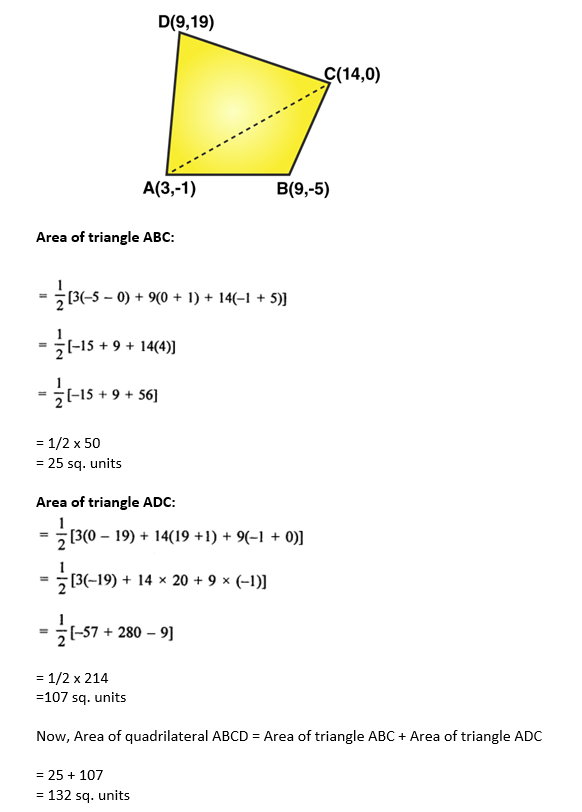Question 3: Find the area of quadrilateral PQRS whose vertices are P (-5, -3), Q (-4, -6), R (2, -3) and S (1, 2).

Solution:

Given: PQRS is a quadrilateral whose vertices are P(-5, -3), Q(-4, -6), R(2, -3) and S(1, 2)

Construction: Join PR

We know that:

$Area of \bigtriangleup ABC=\frac{1}{2}\left [ x_{1}\left ( y_{2} – y_{3}\right ) + x_{2} \left( y_{3} – y_{1}\right )+ x_{3}\left( y_{1} – y_{2}\right )\right ]$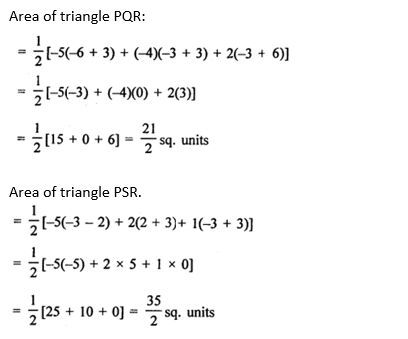Now, Area of quadrilateral PQRS = Area of triangle PQR + Area of triangle PSR

= 21/2 + 35/2

= 28 sq. units

Question 4: Find the area of quadrilateral ABCD whose vertices are A (-3, -1), B (-2, -4), C (4, -1) and D (3, 4).

Solution:

Given: ABCD is a quadrilateral whose vertices are A (-3, -1), B (-2, -4), C (4, -1) and D (3, 4).

By Joining AC, we get two triangles ABC and ADC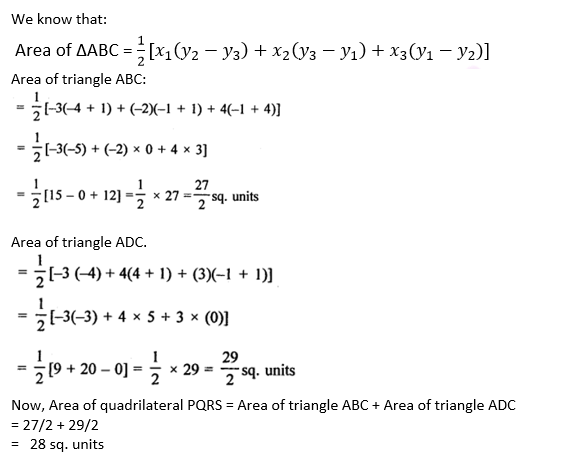Question 5: If A (-7, 5), B (-6, -7), C (-3, -8) and D (2, 3) are the vertices of a quadrilateral ABCD then find the area of the quadrilateral.

Solution: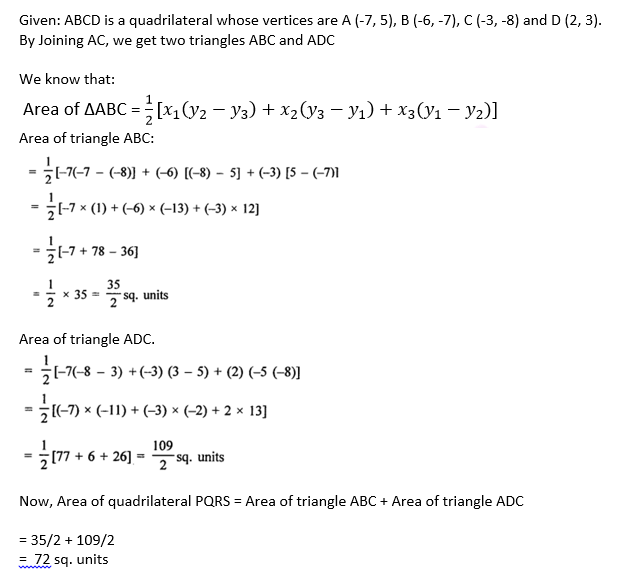Question 6: Find the area of the triangle formed by joining the midpoints of the sides of the triangle whose vertices are A(2, 1), B(4, 3) and C(2, 5).

Solution:

Given: A triangle whose vertices are A(2, 1), B(4, 3) and C(2, 5)

Let D, E and F are the midpoints of the sides CB, CA and AB respectively of ∆ABC, as shown in the below figure.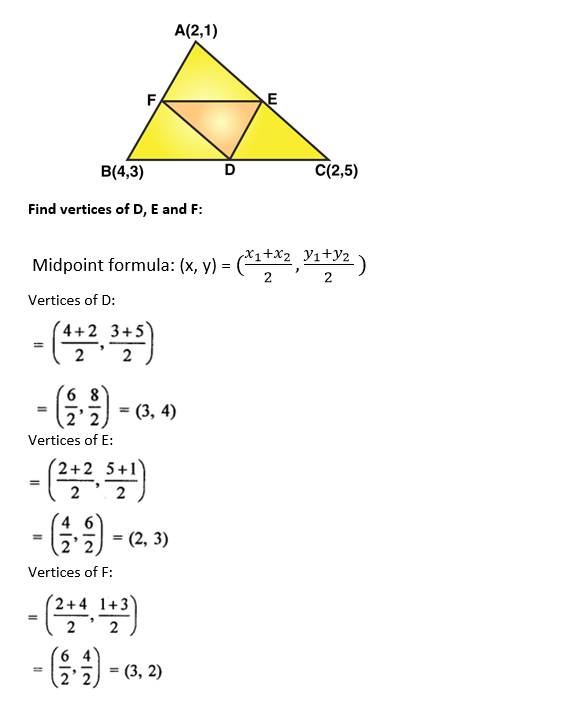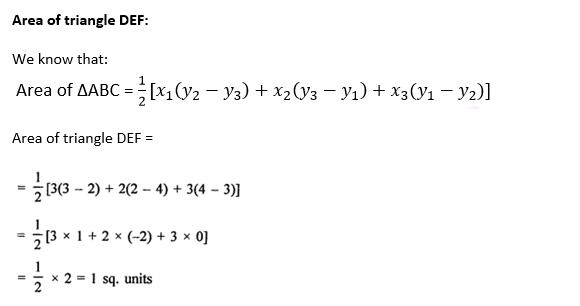Question 7: A (7, -3), B (5, 3) and C (3, -1) are the vertices of a ∆ABC and AD is its median. Prove that the median AD divides ∆ABC into two triangles of equal areas.

Solution: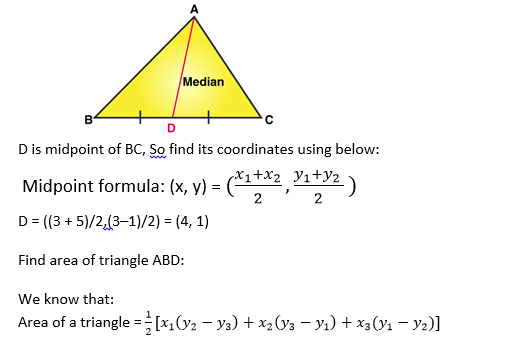So,

Area of triangle ABD:

= 1/2(7(3–1) + 5(1+3) + 4(–3–3))

= 1/2(14 + 20–24)

= 1/2(10)

= 5 sq. units …(1)

Area of triangle ACD:

= 1/2(7(–1–1) + 3(1 + 3) + 4(–3 + 1))

= 1/2(–14 + 12–8)

= 1/2(10)

= 5 sq. units ….(2)

From (1) and (2), we conclude that Area of triangle ABD and ACD is equal.

Hence proved.

Question 8: Find the area of ∆ABC with A (1, -4) and midpoints of sides through A being (2, -1) and (0, -1).

Solution:

Given: A ∆ABC with A(1, -4)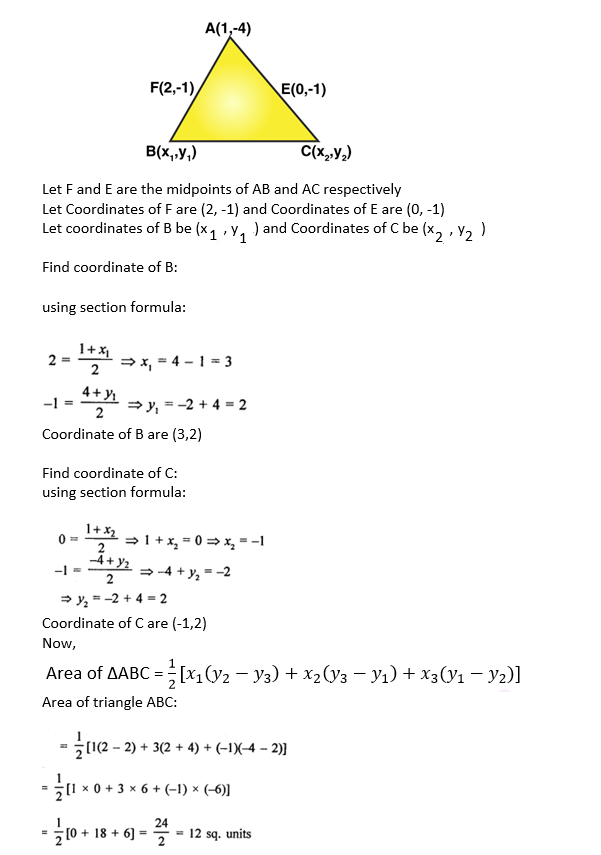Question 9: A(6, 1), B(8, 2) and C(9, 4) are the vertices of a parallelogram ABCD. If E is the midpoint of DC, find the area of ∆ADE.

Solution:

A(6, 1), B(8, 2) and C(9, 4) are the three vertices of a parallelogram ABCD.

E is the midpoint of DC.

Join AE, AC and BD which intersects at O, where O is midpoint of AC.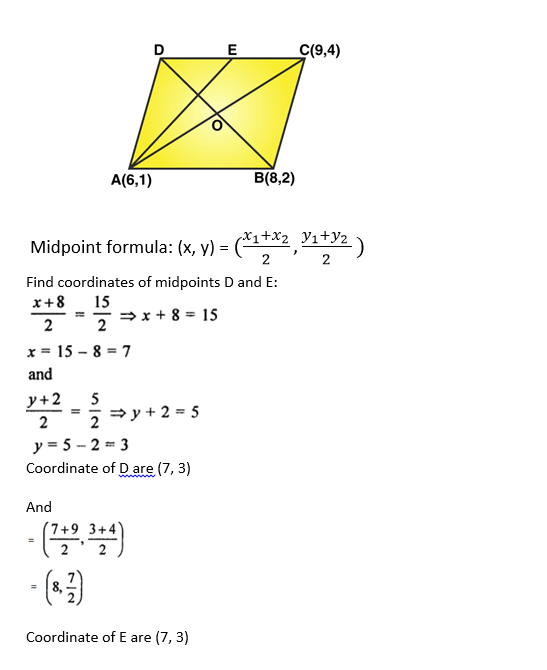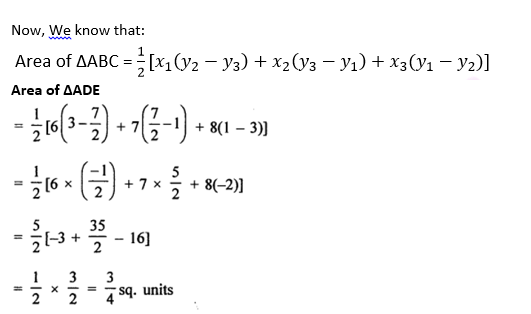Question 10: (i) If the vertices of ∆ABC be A (1, -3), B (4, p) and C (-9, 7) and its area is 15 square units, find the values of p.

(ii) The area of a triangle is 5 sq units. Two of its vertices are (2, 1) and (3, -2). If the third vertex is

(7/2, y), find the value of y.

Solution:

We know that: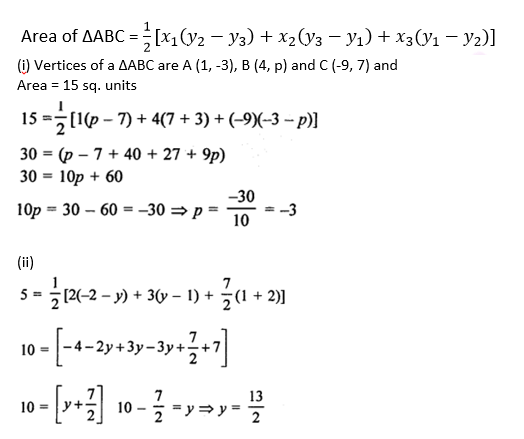Question 11: Find the value of k so that the area of the triangle with vertices A (k + 1, 1), B (4, -3) and C (7, -k) is 6 square units.

Solution: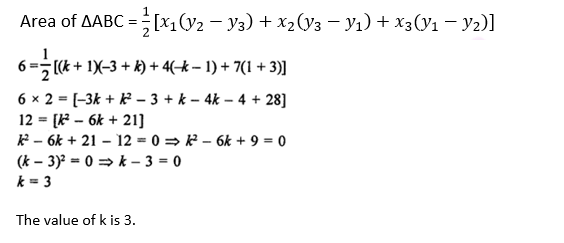Question 12: For what value of k (k > 0) is the area of the triangle with vertices (-2, 5), (k, -4) and (2k + 1, 10) equal to 53 square units?

Solution: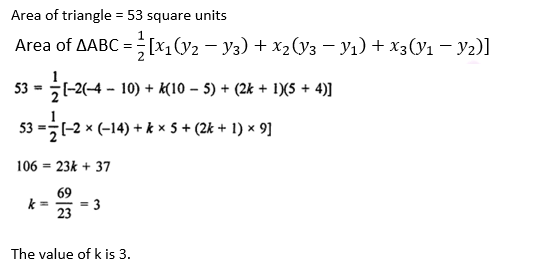Question 13: Show that the following points are collinear.

(i) A (2, -2), B (-3, 8) and C (-1, 4)

(ii) A (-5, 1), B (5, 5) and C (10, 7)

(iii) A (5, 1), B (1, -1) and C (11, 4)

(iv) A (8, 1), B (3, -4) and C (2, -5)

Solution:

Points are collinear if the area of a triangle is equal to zero.

$Area of \bigtriangleup ABC=\frac{1}{2}\left [ x_{1}\left ( y_{2} – y_{3}\right ) + x_{2} \left( y_{3} – y_{1}\right )+ x_{3}\left( y_{1} – y_{2}\right )\right ]$

(i) A (2, -2), B (-3, 8) and C (-1, 4)

Δ = 1/2{2 (8– 4) + (–3) (4 + 2) –1 (2– 8)}

Δ = 1/2 {8–18 + 10}

Δ = 0

Hence points are collinear.

(ii) A (-5, 1), B (5, 5) and C (10, 7)

Δ = 1/2{–5(5– 7) + 5 (7–1) + 10 (1–5)}

Δ = 1/2{10 + 30–40}

Δ = 0

Hence points are collinear.

(iii) A (5, 1), B (1, -1) and C (11, 4)

Δ = 1/2{5(–1– 4) + 1 (4– 1) + 11 (1 + 1)}

= 1/2{–25 + 3 + 22}

= 0

Hence points are collinear.

(iv) A (8, 1), B (3, -4) and C (2, -5)

Δ = 1/2{8(–4 + 5) + 3 (–5–1) + 2 (1 + 4)}

= 1/2{8–18 + 10}

= 0

Hence points are collinear.

Question 14: Find the value of x for which the points A (x, 2), B (-3, -4) and C (7, -5) are collinear.

Solution:

Points are A (x, 2), B (-3, -4) and C (7, -5) are collinear.

Which means area of triangle ABC = 0

$Area of \bigtriangleup ABC=\frac{1}{2}\left [ x_{1}\left ( y_{2} – y_{3}\right ) + x_{2} \left( y_{3} – y_{1}\right )+ x_{3}\left( y_{1} – y_{2}\right )\right ]$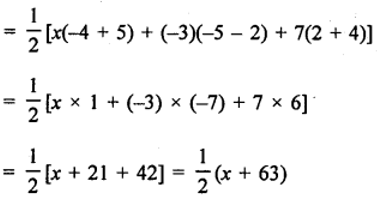Since points are collinear:

½(x + 63) = 0

Or x = -63

Question 15: For what value of x are the points A (-3, 12), B (7, 6) and C (x, 9) collinear?

Solution:

Points are A (-3, 12), B (7, 6) and C (x, 9) are collinear.

Which means area of triangle ABC = 0

$Area of \bigtriangleup ABC=\frac{1}{2}\left [ x_{1}\left ( y_{2} – y_{3}\right ) + x_{2} \left( y_{3} – y_{1}\right )+ x_{3}\left( y_{1} – y_{2}\right )\right ]$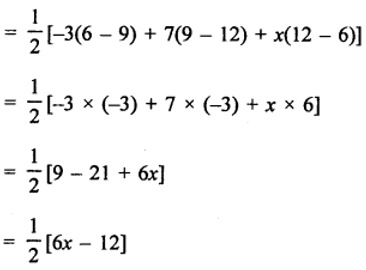Since points are collinear:

½(6x -12) = 0

Or x = 2

Question 16: For what value of y are points P (1, 4), Q (3, y) and R (-3, 16) are collinear?

Solution:

Points are P (1, 4), Q (3, y) and R (-3, 16) are collinear.

Which means area of triangle PQR = 0

$Area of \bigtriangleup ABC=\frac{1}{2}\left [ x_{1}\left ( y_{2} – y_{3}\right ) + x_{2} \left( y_{3} – y_{1}\right )+ x_{3}\left( y_{1} – y_{2}\right )\right ]$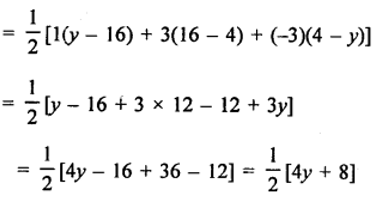Since points are collinear:

½(4y + 8) = 0

Or y = -2

Question 17: Find the value of y for which the points A (-3, 9), B (2, y) and C (4, -5) are collinear.

Solution:

Points are A (-3, 9), B (2, y) and C (4, -5) are collinear.

Which means area of triangle ABC = 0

$Area of \bigtriangleup ABC=\frac{1}{2}\left [ x_{1}\left ( y_{2} – y_{3}\right ) + x_{2} \left( y_{3} – y_{1}\right )+ x_{3}\left( y_{1} – y_{2}\right )\right ]$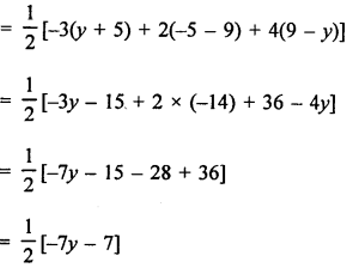Since points are collinear:

½(-7y – 7) = 0

Or y = -1

Question 18: For what values of k are the points A (8, 1), B (3, -2k) and C (k, -5) collinear.

Solution:

Points are A (8, 1), B (3, -2k) and C (k, -5) are collinear.

Which means area of triangle ABC = 0

$Area of \bigtriangleup ABC=\frac{1}{2}\left [ x_{1}\left ( y_{2} – y_{3}\right ) + x_{2} \left( y_{3} – y_{1}\right )+ x_{3}\left( y_{1} – y_{2}\right )\right ]$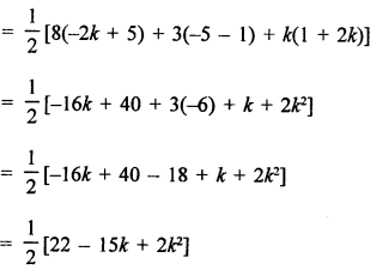Since points are collinear:

½(2k^2 – 15k + 22) = 0

Or 2k^2 – 15k + 22 = 0

2k^2 – 11k – 4k + 22 = 0

k(2k – 11) – 2(2k – 11) = 0

(k-2)(2k -11) = 0

k = 2 or k = 11/2. Answer.

Question 19: Find a relation between x and y, if the points A(2, 1), B(x, y) and C(7, 5) are collinear.

Solution:

Points are A(2, 1), B(x, y) and C(7, 5) are collinear.

Which means area of triangle ABC = 0

$Area of \bigtriangleup ABC=\frac{1}{2}\left [ x_{1}\left ( y_{2} – y_{3}\right ) + x_{2} \left( y_{3} – y_{1}\right )+ x_{3}\left( y_{1} – y_{2}\right )\right ]$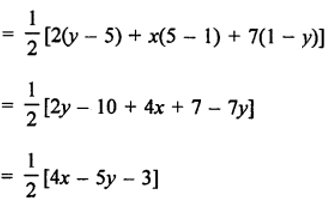Since points are collinear:

½(4x -5y – 3) = 0

4x – 5y – 3 = 0

Relationship between x and y.

Question 20: Find a relation between x and y, if the points A(x, y), B(-5, 7) and C(-4, 5) are collinear.

Solution:

Points are A(x, y), B(-5, 7) and C(-4, 5) are collinear.

Which means area of triangle ABC = 0

$Area of \bigtriangleup ABC=\frac{1}{2}\left [ x_{1}\left ( y_{2} – y_{3}\right ) + x_{2} \left( y_{3} – y_{1}\right )+ x_{3}\left( y_{1} – y_{2}\right )\right ]$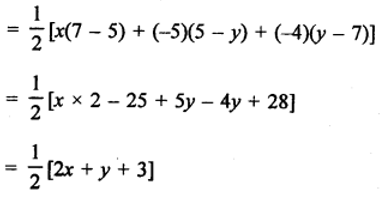Since points are collinear:

½(2x +y + 3) = 0

2x +y + 3 = 0

Relationship between x and y.

Question 21: Prove that the points A(a, 0), B(0, b) and C(1, 1) are collinear, if 1/a + 1/b = 1.

Solution:

Points are A(a, 0), B(0, b) and C(1, 1) are collinear.

Which means area of triangle ABC = 0

$Area of \bigtriangleup ABC=\frac{1}{2}\left [ x_{1}\left ( y_{2} – y_{3}\right ) + x_{2} \left( y_{3} – y_{1}\right )+ x_{3}\left( y_{1} – y_{2}\right )\right ]$

= ½[(a(b-1) + 0(1-0) + 1(0-b)]

= ½[ab – a + 0 – b]

Since points are collinear:

½(ab -a-b) = 0

ab – a – b = 0

Divide each term by “ab”, we get

1 – 1/b -1/a = 0

or 1/a + 1/b = 1. hence proved.

Question 22: If the points P(-3, 9), Q(a, b) and R(4, -5) are collinear and a + b = 1, find the values of a and b.

Solution:

Points are P(-3, 9), Q(a, b) and R(4, -5) are collinear.

Which means area of triangle = 0

$Area of \bigtriangleup ABC=\frac{1}{2}\left [ x_{1}\left ( y_{2} – y_{3}\right ) + x_{2} \left( y_{3} – y_{1}\right )+ x_{3}\left( y_{1} – y_{2}\right )\right ]$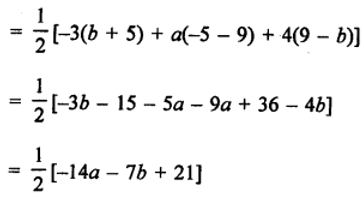Since points are collinear, we have

½(-14a – 7b + 21) = 0

-14a – 7b + 21 = 0

2a + b =3 …..(1)

a + b = 1 …..(2) (given)

From (1) and (2)

a = 2 and b = -1

Question 23: Find the area of ∆ABC with vertices A (0, -1), B (2, 1) and C (0, 3). Also, find the area of the triangle formed by joining the midpoints of its sides. Show that the ratio of the areas of two triangles is 4 : 1.

Solution:

Vertices of ∆ABC are A (0, -1), B (2, 1) and C (0, 3)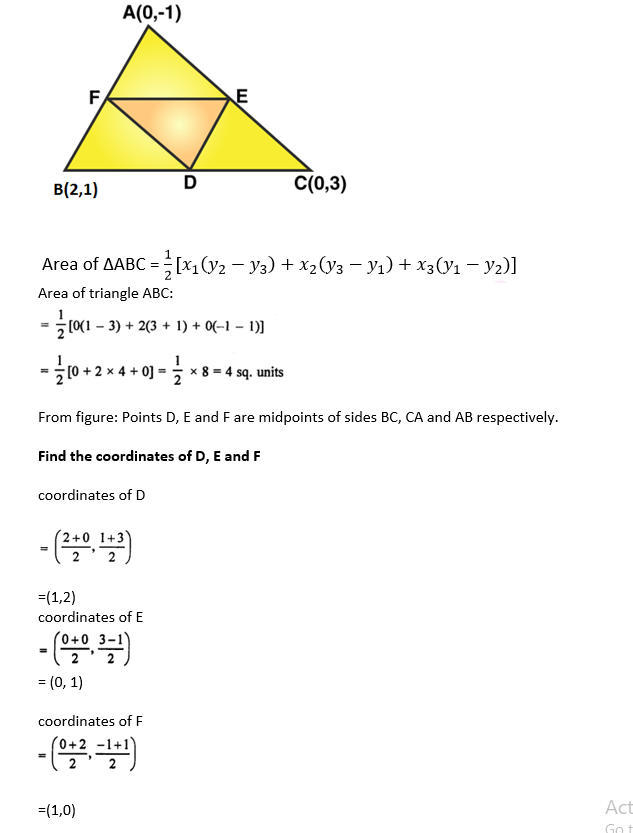Area of triangle DEF:

= 1/2[1+0+1]

= 1 sq. units

Therefore,

Ratio in the area of triangles ABC and DEF = 4/1 = 4:1.

Question 24: If a ≠ b ≠ c, prove that (a, a²), (b, b²), (0, 0) will not be collinear.

Solution:

Let A (a, a²), B (b, b²) and C (0, 0) are the vertices of a triangle.

Let us assume that that points are collinear, then area of ∆ABC must be zero.

Now, area of ∆ABC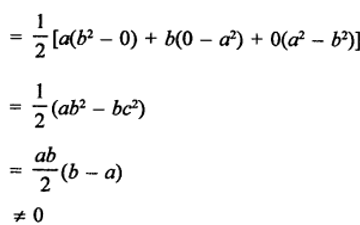Which is contraction to our assumption.

This implies points are not be collinear. Hence proved.

## R S Aggarwal Solutions for Class 10 Maths Chapter 6 Coordinate Geometry Exercise 6C Topics

Class 10 Maths Chapter 6 Coordinate Geometry Exercise 6C is based on following topics:

• Area of a triangle

Area of ∆ABC whose vertices are is (x1,y1 ),(x2,y2 )and (x3,y3 ) are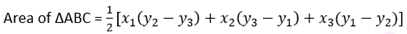Students can also find the collinearity of three points using area of triangle formula.